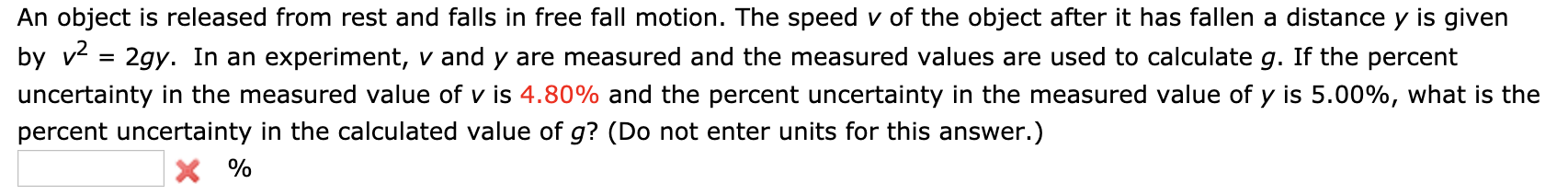# An object is released from rest and falls in free fall motion. The speed v of the object after it has fallen a distance y is givenby v2gy. In an experiment, v and y are measured and the measured values are used to calculate g. If the percentuncertainty in the measured value of v is 4.80% and the percent uncertainty in the measured value of y is 5.00%, what is thepercent uncertainty in the calculated value of g? (Do not enter units for this answer.)X%

Question
3 viewshelp_outlineImage TranscriptioncloseAn object is released from rest and falls in free fall motion. The speed v of the object after it has fallen a distance y is given by v 2gy. In an experiment, v and y are measured and the measured values are used to calculate g. If the percent uncertainty in the measured value of v is 4.80% and the percent uncertainty in the measured value of y is 5.00%, what is the percent uncertainty in the calculated value of g? (Do not enter units for this answer.) X% fullscreen
check_circle

Step 1

The expression for the speed of the object  in terms of distance and the acceleration due to gravity  is given as,

Step 2

Use propagation uncertainty equatio...

### Want to see the full answer?

See Solution

#### Want to see this answer and more?

Solutions are written by subject experts who are available 24/7. Questions are typically answered within 1 hour.*

See Solution
*Response times may vary by subject and question.
Tagged in

### Physics